Question

# Problem 2 An RC circuit ( with an active component) has the following transfer function (where...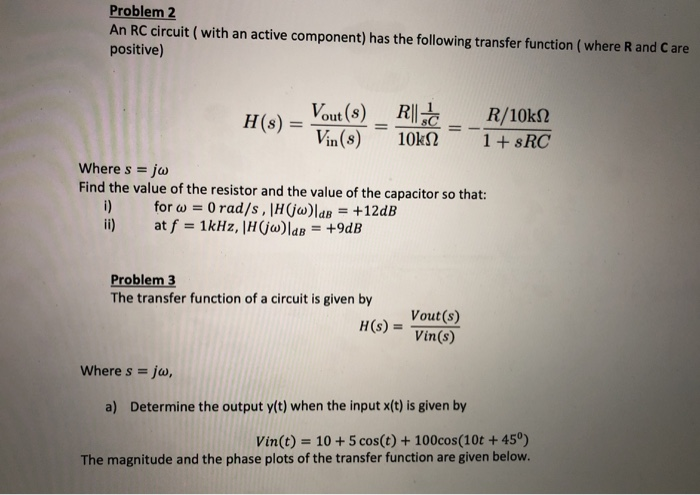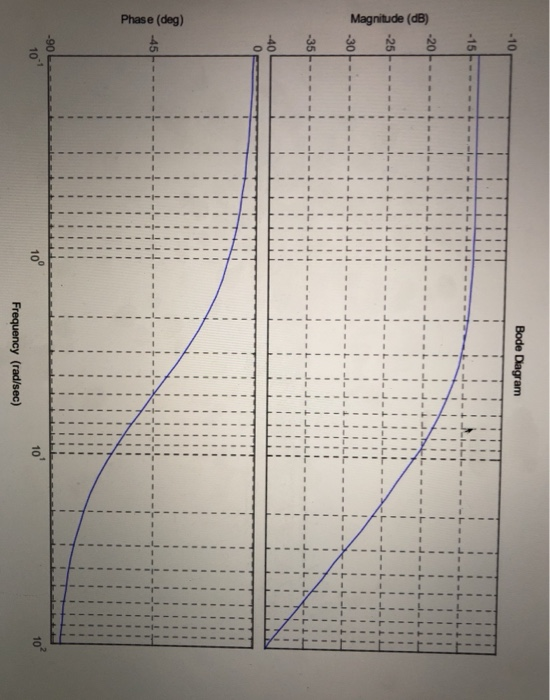Problem 2 An RC circuit ( with an active component) has the following transfer function (where R and Care positive) H(s) - Vout(8) _R|| R/10k12 Vin(8) 10KN 1 + \$RC Where s = jw Find the value of the resistor and the value of the capacitor so that: for w = 0 rad/s, H(jw)lde = +12dB at f = 1kHz, |H(jw)lab = +9dB Problem 3 The transfer function of a circuit is given by H(S) = Vout(s) Vin(s) Where s = jw, a) Determine the output y(t) when the input x(t) is given by Vin(t) = 10 + 5 cos(t) + 100cos(10t + 45°) The magnitude and the phase plots of the transfer function are given below.
Bode Diagram Magnitude (dB) 1 1 1 Phase (deg) 101 Frequency (rad/sec)

We need at least 10 more requests to produce the answer.

0 / 10 have requested this problem solution

The more requests, the faster the answer.

All students who have requested the answer will be notified once they are available.

#### Earn Coins

Coins can be redeemed for fabulous gifts.

Similar Homework Help Questions
• ### QUESTION #2 PLEASE 1. Derive the transfer function for the circuit shown below. Plot H(s) versus...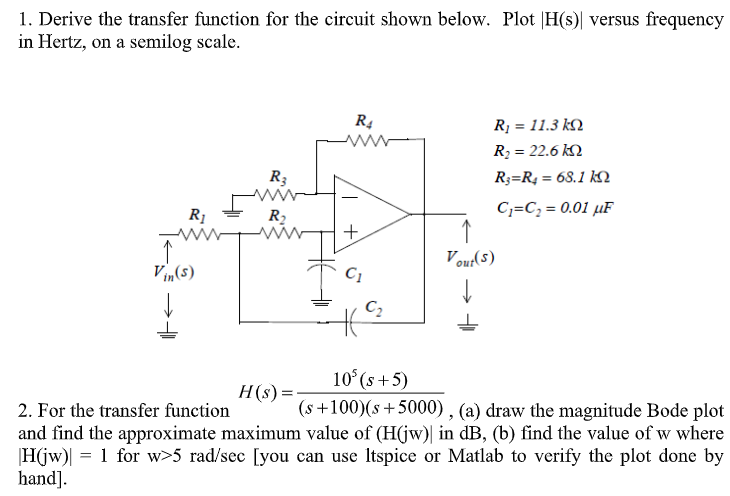QUESTION #2 PLEASE 1. Derive the transfer function for the circuit shown below. Plot H(s) versus frequency in Hertz, on a semilog scale. Ri 11.3 k Ri 22.6 k R R = 68.1 kN R3 C C 0.01 uF R2 Vout(s) Vin(s) C2 10 (s+5) H(s) = (s+100)(s5000) , (a) draw the magnitude Bode plot 2. For the transfer function and find the approximate maximum value of (H(jw) in dB, (b) find the value of w where 1 for w>5...

• ### (5 points) You are given a circuit with the following transfer 5jw function, H(jw) = (jw+2)(0.2.jw+1)...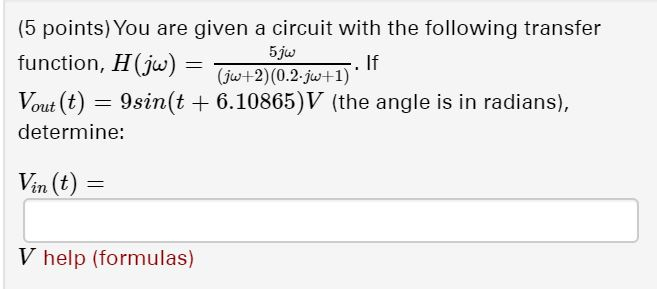(5 points) You are given a circuit with the following transfer 5jw function, H(jw) = (jw+2)(0.2.jw+1) Vout (t) = 9sin(t + 6.10865)V (the angle is in radians), determine: Vin (t) = V help (formulas)

• ### Find the transfer function H(s) for the circuit below. Make s=jw and plot the Bode plot...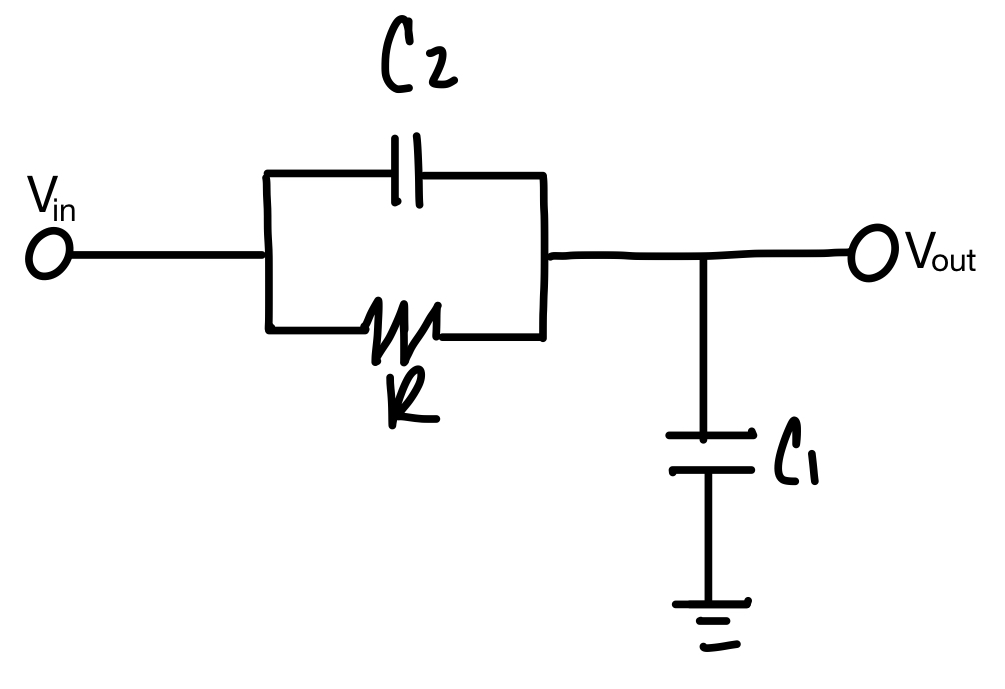Find the transfer function H(s) for the circuit below. Make s=jw and plot the Bode plot (magnitude and phase response) for the transfer function. C = out 페

• ### A bode plot of the transfer function, GS = - 25 \$2+45+25, is shown as below....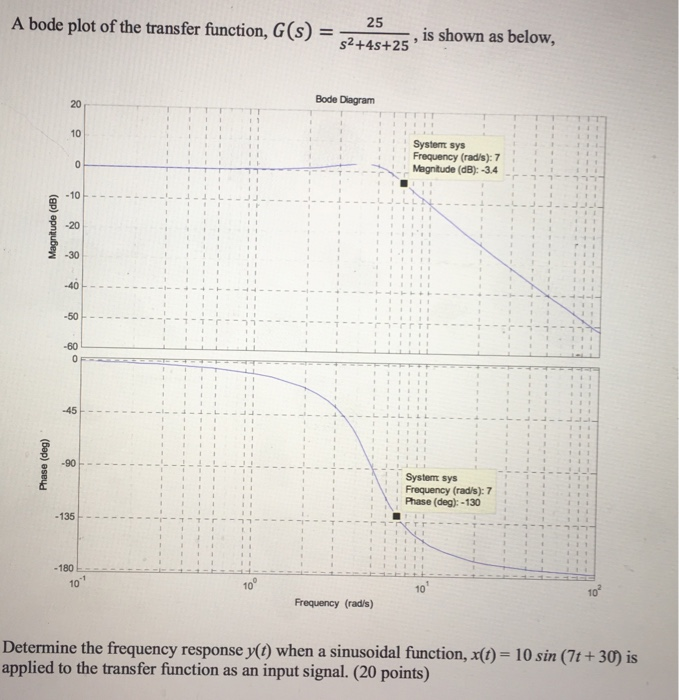A bode plot of the transfer function, GS = - 25 \$2+45+25, is shown as below. Bode Diagram System sys Frequency (rad/s): 7 Magnitude (dB): -3.4 Magnitude (dB) Phase (deg) Systemt sys Frequency (rad/s): 7 Phase (deg): - 130 - 135 - 180 10 Frequency (rad/s) Determine the frequency response y(t) when a sinusoidal function, X(t) = 10 sin (7t +30) is applied to the transfer function as an input signal. (20 points)

• ### For the circuit shown below (1) Please derive an expression for the transfer function H(jco)- Vout/Vin....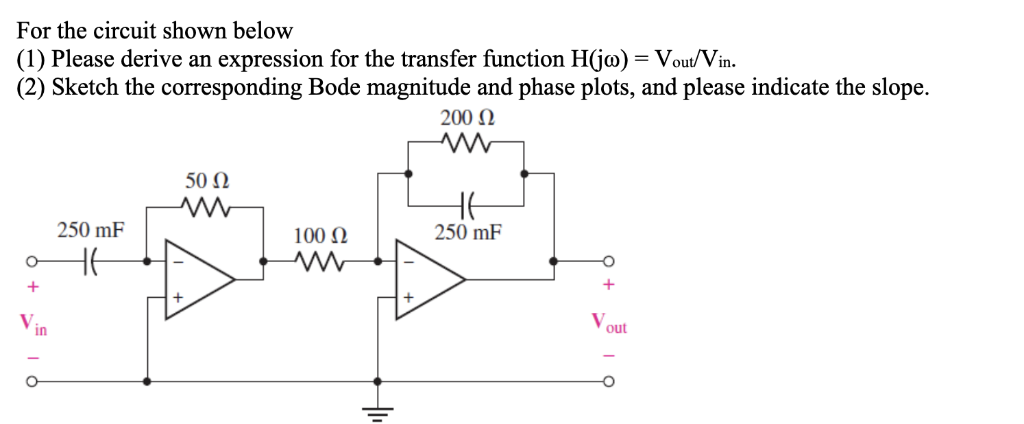For the circuit shown below (1) Please derive an expression for the transfer function H(jco)- Vout/Vin. (2) Sketch the corresponding Bode magnitude and phase plots, and please indicate the slope. 50Ω 250 mF 100 Ω 250 mF in out

• ### Show clear work, please and thank you! Will upvote. 4. (40 pts total, 10 pts per...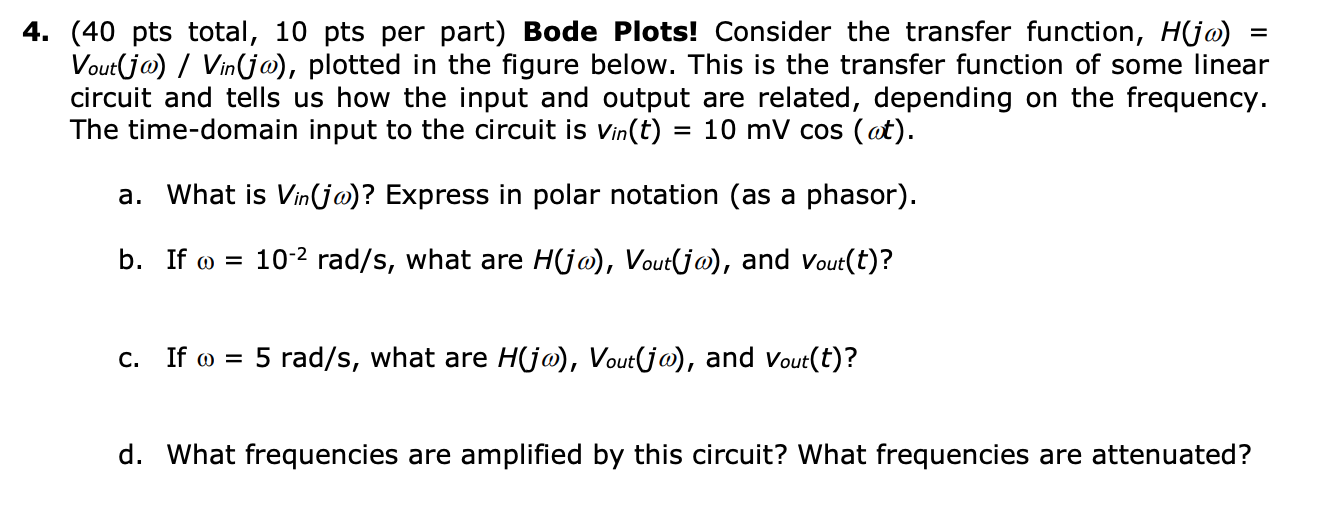Show clear work, please and thank you! Will upvote. 4. (40 pts total, 10 pts per part) Bode Plots! Consider the transfer function, H(ja) Vout(ja) Vinjo), plotted in the figure below. This is the transfer function of some linear circuit and tells us how the input and output are related, depending on the frequency. The time-domain input to the circuit is vin(t) = 10 mV cos (at). a. What is Vin(jo)? Express in polar notation (as a phasor) b. If...

• ### Please answer all parts Problem #5 - (20%) A circuit has the transfer function: H(S) =...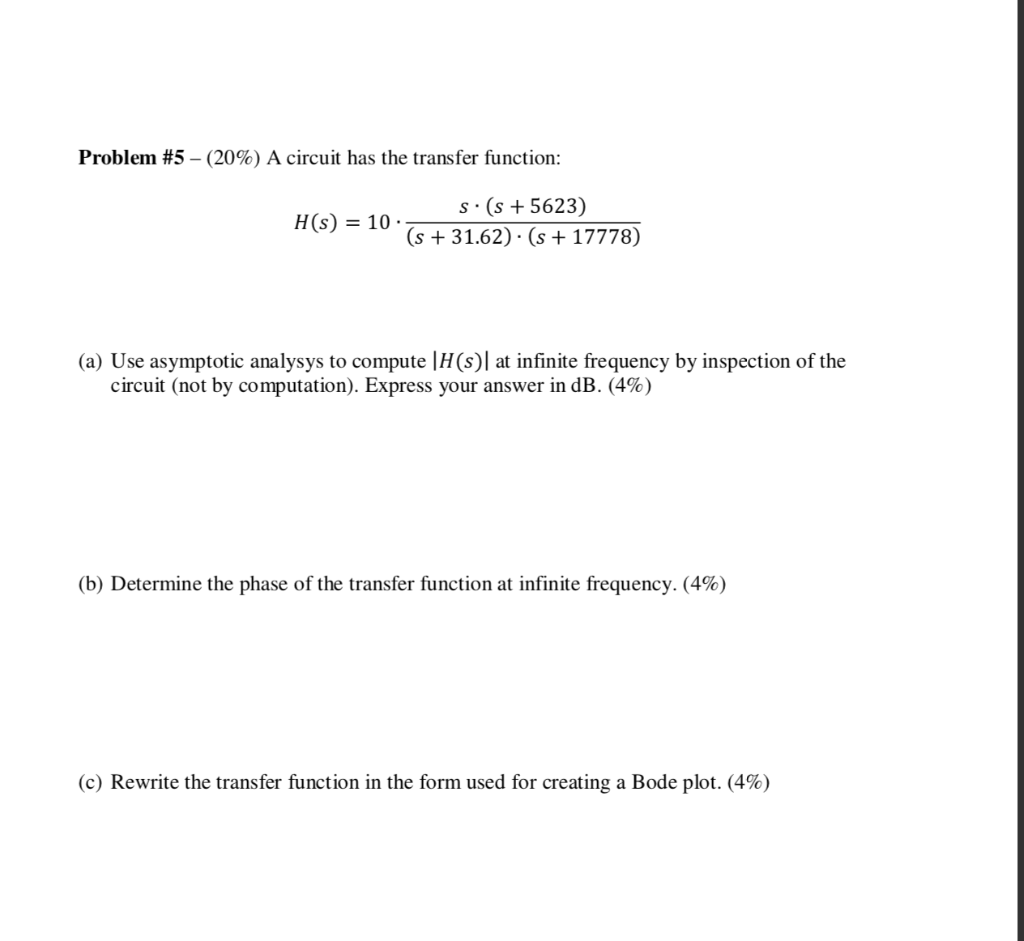Please answer all parts Problem #5 - (20%) A circuit has the transfer function: H(S) = S. (s + 5623 (s + 31.62) · (s + 17778) (a) Use asymptotic analysys to compute (HS) at infinite frequency by inspection of the circuit (not by computation). Express your answer in dB. (4%) (b) Determine the phase of the transfer function at infinite frequency. (4%) (c) Rewrite the transfer function in the form used for creating a Bode plot. (4%) Problem #5...

• ### Find the transfer function T(jw) for the circuit below. Also find the magnitude response, 0 OP000...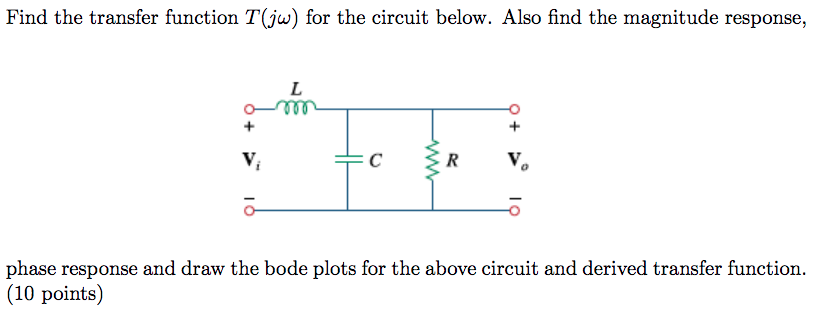Find the transfer function T(jw) for the circuit below. Also find the magnitude response, 0 OP000 + + C R 1 phase response and draw the bode plots for the above circuit and derived transfer function. (10 points)

• ### etermine the transfer function of the circuit where i^(t) is the output variable and v.ct) is...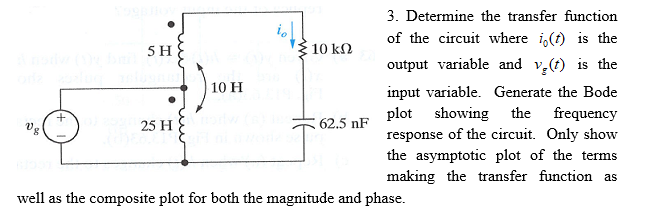etermine the transfer function of the circuit where i^(t) is the output variable and v.ct) is the input variable. Generate the Bode plot showing the frequency response of the circuit. Only show the asymptotic plot of the terms making the transfer function as 5 H 10 k2 10 H 25 H 62.5 nF U) well as the composite plot for both the magnitude and phase.

• ### Problem 5: (20 points) Given the following circuit VIN L Vout R a) Write the loop...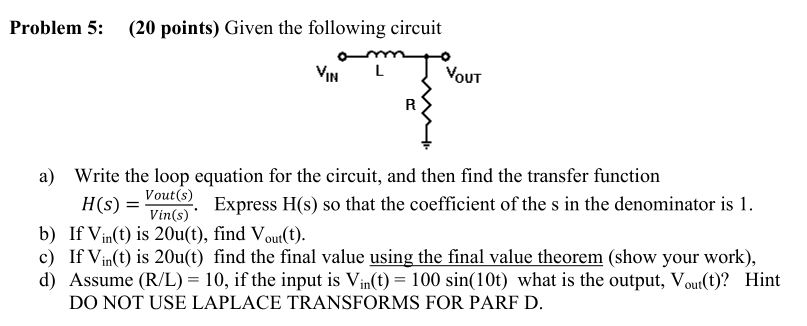Problem 5: (20 points) Given the following circuit VIN L Vout R a) Write the loop equation for the circuit, and then find the transfer function Vout(s) H(S) = Express H(s) so that the coefficient of the s in the denominator is 1. Vin(s) b) If Vin(t) is 20u(t), find out(t). c) If Vin(t) is 20u(t) find the final value using the final value theorem (show your work), d) Assume (R/L) = 10, if the input is Vin(t) = 100...